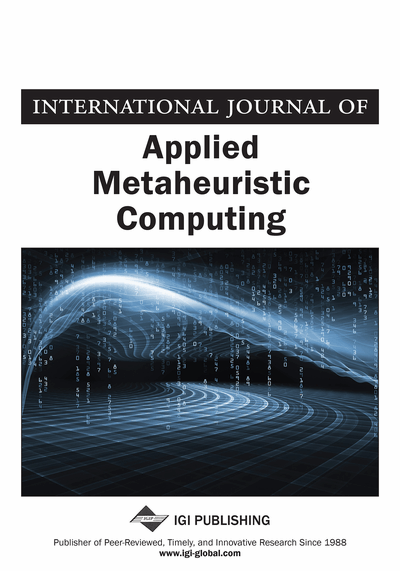# Reliability Analysis of Circular Footing by Using GP and MPMR

Rahul Kumar (National Institute of Technology, Patna, India), Pijush Samui (National Institute of Technology, Patna, India), Sunita Kumari (National Institute of Technology, Patna, India) and Yildirim Hüseyin Dalkilic (Erzincan Binali Yıldırım University, Turkey)
DOI: 10.4018/IJAMC.2021010101
Available
\$37.50
No Current Special Offers

## Abstract

Circular footings are designed to bear a load of super structures. Studies have been done on the influence of soil properties on bearing capacity of shallow foundations. The use of circular foundation is practical in geotechnical engineering. During the design of circular footing, bearing capacity of soil is taken into consideration, and cohesion (c), unit weight (γ), and angle of internal friction (ϕ) are the most variable parameters. Reliability analysis is used frequently for the design of circular footing. Most of the authors have used first order second moment methods (FOSM). However, FOSM is a time-consuming method. Drawbacks of FOSM have been overcome by genetic programming (GP), minimax probability machine regression (MPMR). This article gives a distinct analysis between the developed MPMR based FOSM and GP-based FOSM.
Article Preview
Top

## 1. Introduction

While the analysis of circular footing, Geotechnical engineers often face with the problem of the error of the parameters (i.e. c, ϕ and γ) of soil. Because of the error in the parameters, exact results are not obtained. So, in order to improve the design, reliability analysis has to be used. The sortilege of performance of numerical model is affected by uncertainty.Reliability analysis minimises the error of the parameters. Reliability analysis of circular footing has been done by FOSM(Alawneh, Nusier, & Al-Mufty, 2006; Cherubini, 2000; Guan & Melchers, 1997; Honjo, Suzuki, & Matsuo, 2000; Phoon, Kulhawy, & Grigoriu, 2003; Priyadarshini & Mishra, 2017). However, FOSM has some drawbacks as it is time-consuming for implicit performance function. Various researchers used different modelse.g. ANN Particle swarm optimization.(PSO), Artificial neural network (ANN), Response surface method (RSM) (Bucher & Bourgund, 1990; Faravelli, 1989; Rajashekhar & Ellingwood, 1993; Wong, 1985), multi-plane surface to overcome the above drawbacks of FOSM .For the reliability analysis of ultimate bearing capacity of circular footing, two models GP & MPMR have been adopted. GP is the expansion of the genetic algorithm (Koza, 1992). GP is a set of methods for the automatic generation of computer programs that solve carefully stated problems on the principles of natural selection. It is the composite breeding of (initially random) computer programs, where only the comparatively more effectiveentities pass on genetic material to the following output.

MPMR depends on Minimax Probability Machine Classification (MPMC) (Lanckriet, El Ghaoui, Bhattacharyya, & Jordan, 2003).It make the most of the minimum probability that forecast of an output of the regression model is within certain conditions of the true regression function. The basic framework of MPMR is that its true regression function considers the minimal assumptions about underlying distribution, because estimation of these distributions is prohibitive for most trivial problem especially for regression (Kumar, Samui, & Naithani, 2013).

In this article, ultimate bearing capacity (qu) of a circular footing has been calculated by using GP and MPMR. For the computation of qu different parameters have been taken which are as: c, ϕ, γ, diameter of circular footing (D) and depth of the footing (Df). To calculate the qu, Terzaghi bearing capacity of circular footing Eq. 1 has been used(Arora, 2004).

(1) Where, B is the diameter of the footing, Nc, Nq, and Nγ are the general bearing capacity factor, ‘Df’ is the depth of foundation, ‘γ’ is the unit weight of the soil and ‘c’ is the cohesion of soil.

• To find the reliability index (β) of circular footing by using GP based FOSM and MPMR based FOSM methods.

• To make the relativeanalysis between the developed GP based FOSM and MPMR based FOSM approaches.

Top

## 2. Detailsof Mpmr

MPMR is based on kernel formulation (Lanckriet et al., 2003). This paragraph gives the details about MPMR for prediction of bearing capacity of circular footing. To solve the regression problems, MPMR follows Eq. 2.

(2) where y is output, x is input, N is number of datasets, K(xi,x) is kernel function, βi and b are output of the MPMR algorithm. This study uses the c, Φ,γ as the input. So, x= [c, ϕ, γ] and y=[qu].

Various scholars have used MPMR in different areas (Samui & Hariharan, 2014; Samui, Kim, & Hariharan, 2015; Viswanathan, Jagan, Samui, & Porchelvan, 2015).In this article MPMR uses Radial basis kernel function which value is 0.5 and error insensitive zone is 0.003. For the determining the qu, 80 random values of input which soil parameters are taken. On the basis of above random valuedatasets created, which is given in Table1, β has been calculated.

Table 1 shows the dataset of circular footing in which c, ϕ, γ and qu are given.

## Complete Article List

Search this Journal:
Reset
Volume 13: 4 Issues (2022): 2 Released, 2 Forthcoming
Volume 12: 4 Issues (2021)
Volume 11: 4 Issues (2020)
Volume 10: 4 Issues (2019)
Volume 9: 4 Issues (2018)
Volume 8: 4 Issues (2017)
Volume 7: 4 Issues (2016)
Volume 6: 4 Issues (2015)
Volume 5: 4 Issues (2014)
Volume 4: 4 Issues (2013)
Volume 3: 4 Issues (2012)
Volume 2: 4 Issues (2011)
Volume 1: 4 Issues (2010)
View Complete Journal Contents Listing